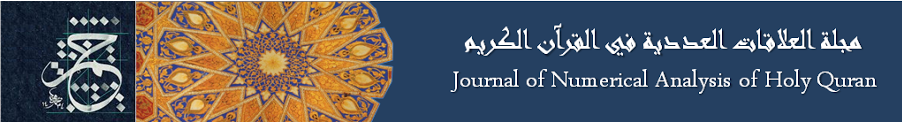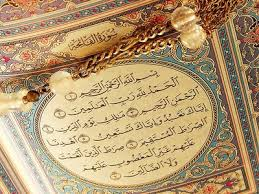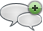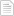### A note about 7^n + 29^n + 139^n

#### الجمعة، 17 إبريل 2015 - 1:50(Regarding the numbers in Surat Alfateha:  7 ayats, 29 words, 139 letters)

Theorem:

7n + 29n + 139n is always a composite number for every n. Proof. Note that 29 + 139 = 168 = 7 × 24, hence for odd number n, 29n + 139n has a factor 29 + 139. Thus the result holds. So we assume n = 2k.Let

φ(k) = 72k + 292k + 1392k = 49k + 841k + 19321k .

Note that

49 ≡ 1(mod 3),

841 ≡ 1(mod 3),

19321 ≡ 1(mod 3),

then

49k ≡ 1(mod 3),

841k ≡ 1(mod 3),

19321k ≡ 1(mod 3).

Therefore, 49k + 841k + 19321k ≡ 3(mod 3), that is 3|49k + 841k + 19321k .أضف تعليقك
##### رجاء ضرورة الإلتزام بعدم إضافة أي تعليق يمس أو يسيء للأديان أو المعتقدات أو المقدسات, كما نرجو ألا يتضمن التعليق السباب أو أي ألفاظ تخدش الحياء والذوق العام

 الأكثر قراءةSura Alfati7a, Alfalaq and Annas all use 21 Letters of the Alphabet.النمل.السجدة.حساب الجمّل علم الأنبياء.السور التي يتدأ بــ "يَٰٓأَيُّهَا ٱلَّذِينَ ءَامَنُوٓا۟ " مجموعها 114.Number 114 is an Indication of Completeness.ذكر موسى عليه السلام في القرآن.تعيين ليلة القدر من سورة الدخان.الكهف.ملاحظة في ترتيب سور فصلت والشورى وق.
الموقع الرسمي حم © 2015, كل الحقوق محفوظة |